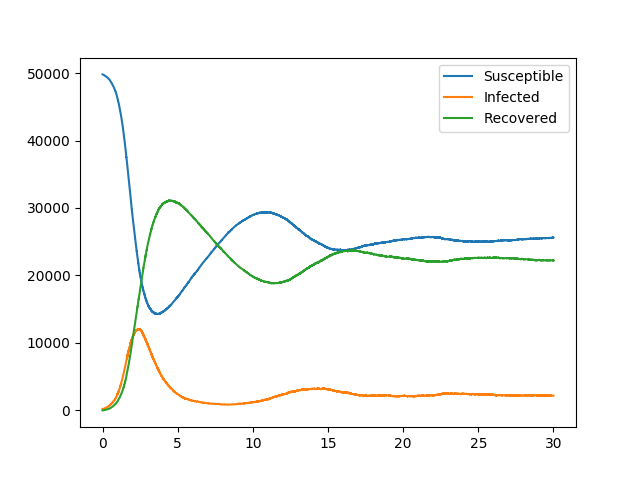SIRS with Heterogeneity¶We consider a model comparable to our basic SIRS example, except that some of the contacts are more infectious than before. However, some individuals recover faster, while others take longer to return to the susceptible state after recovery. So the difference from our basic SIRS outcomes are not very large.

Specifically we have transmission and recovery rates depend on age and gender. Transmission rates are not always symmetric, so it is not as simple as introducing a weight to scale the partnerships. So we introduce functions to scale the transition rates.

The method is built on Gillespie_simple_contagion

import EoN
import networkx as nx
from collections import defaultdict
import matplotlib.pyplot as plt
import random

N = 50000
G = nx.fast_gnp_random_graph(N, 5./(N-1))

#Let's consider a disease like that in the basic SIRS example, except:
#   children are more susceptible
#   males are more infectious if the partner is female
#   children recover faster.
#   and let's say that we want the cutoff age for a child to be a parameter

#So first we define the node attributes:
ages = {node: random.random()*100 for node in G}
genders = {node: 'M' if random.random()<0.5 else 'F' for node in G}
nx.set_node_attributes(G, values=ages, name = 'age')
nx.set_node_attributes(G, values = genders, name = 'gender')

#Now we define functions which will be used to scale the transition rates
def transmission_weighting(G, source, target, **kwargs):
scale = 1
if G.node[target]['age']<kwargs['age_cutoff']:
scale *= 1.5
if G.node[target]['gender'] is 'F' and G.node[source]['gender'] is 'M':
scale *= 1.5
return scale

def recovery_weighting(G, node, **kwargs):
scale = 1
if G.node[node]['age']<kwargs['age_cutoff']:
scale *= 1.5
return scale

def return_to_susceptibility_weighting(G, node, **kwargs):
scale = 1
if G.node[node]['gender'] is 'F':
scale *= 0.5
return scale

H = nx.DiGraph()  #DiGraph showing possible transitions that don't require an interaction
H.add_edge('I', 'R', rate = 1.4, rate_function=recovery_weighting)   #I->R
H.add_edge('R', 'S', rate = 0.2, rate_function = return_to_susceptibility_weighting)   #R->S

J = nx.DiGraph()    #DiGraph showing transition that does require an interaction.
J.add_edge(('I', 'S'), ('I', 'I'), rate = 1, rate_fuction = transmission_weighting)  #IS->II

IC = defaultdict(lambda: 'S')
for node in range(200):
IC[node] = 'I'

return_statuses = ('S', 'I', 'R')

age_cutoff = 18
t, S, I, R = EoN.Gillespie_simple_contagion(G, H, J, IC, return_statuses, tmax = 30,
spont_kwargs = {'age_cutoff':age_cutoff},
nbr_kwargs = {'age_cutoff':age_cutoff})

plt.plot(t, S, label = 'Susceptible')
plt.plot(t, I, label = 'Infected')
plt.plot(t, R, label = 'Recovered')
plt.legend()
plt.savefig('SIRS_heterogeneous.png')